# Vertical Asymptote: Have An Easy Time Finding It!One of the A-words that often send shivers into students’ spines is asymptote. To most college students, ‘asymptote’ is so complex and impossible to solve. However, if you understand the basic concepts and rules, calculating asymptote will not just be easy, but also fun.

There are three main types of asymptote; vertical, horizontal, and oblique. In this post, we are going to focus on the vertical asymptote. We will delve deeper to establish its rules and use examples to demonstrate how to find vertical asymptotes.

## A Closer Look at Vertical Asymptote

A vertical asymptote often referred to as VA, is a vertical line (x=k) indicating where a function f(x) gets unbounded. This implies that the values of y get subjectively big either positively (y→ ∞) or negatively (y→ -∞) when x is approaching k, no matter the direction. When these functions are demonstrated in a graph, they form curves that avoid certain invisible lines (asymptote). You cannot get the graph to cross those lines! See the demonstration of an asymptote in Figure 1.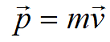Figure 1: A graph demonstrating asymptotes – (Asymptotes are shown with dashed lines. Note the way the graph avoids them).

To help you grasp the concept of asymptote, think of an airplane headed to a huge mountain. If the pilot does not have an option of going left or right to evade the mountain, what option is left? He would consider flying upwards to avoid hitting the mountain. But if the mountain is infinitely high, he would fly vertically forever.

When working on how to find the vertical asymptote of a function, it is important to appreciate that some have many VAs while others don’t. In the demonstration below (figure 2), at point X, there are two asymptotes, X=1 and X=-3.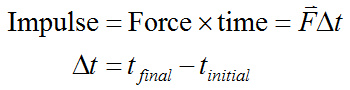Figure 2: A graph showing a function with two asymptotes.

### Vertical Asymptote Rules

When you have a task to find vertical asymptote, it is important to understand the basic rules. Indeed, you can never get it right on asymptotes without grasping these three rules. Therefore, make sure to grasp them well.

1. When the graph gets closer to the vertical asymptote, it tends to negative/ positive infinity. You can see that from both Figure 1 and Figure 2.
2. The distance between asymptote and graph tends to zero when the latter draws near. Looking at the tips from afar, asymptote and graph appear to almost merge. However, the curve does not touch the asymptote. It looks like the asymptote has a sort of a thin barrier that keeps the graph from touching it.
3. The graph, as shown in Figure 1, can approach the asymptote from any direction (right or left). However, it is important to appreciate that there are some functions that can only approach the vertical asymptote from only one direction.

### How to Find Vertical Asymptote

There are two ways on how to find a vertical asymptote in calculus; graphically and analytically.

• Using a graph to find asymptote
When you are presented with a graph, you simply need to look for breaks. If the branch of a specific function changes towards the vertical, it is probably a VA. To know the value of asymptote, consider sketching a line where you think the asymptote should be located. However, you do not have an asymptote if the graph touches your vertical line.
• How to find vertical asymptotes of a function using an equation
A more accurate method of how to find vertical asymptotes of rational functions is using analytics or equation. Here are the two steps to follow. Talking of rational function, we mean this: when f(x) takes the form of a fraction, f(x) = p(x)/q(x), in which q(x) and p(x) are polynomials.

1. Step one: Factor the denominator and numerator. This is crucial because if both factors on each end cancel out, they cannot form a vertical asymptote.
2. Step two: After reducing the rational fraction, take a closer look at the denominator to establish its factors. If any of the factors on the denominator involve (x-a), ­it means that x=a is a vertical asymptote. Again, if you have any factor that involves (x+a), it means that x=-a is a vertical asymptote. If you take a closer look, you will realize that the signs appear to be the opposite.

### How to Find Asymptotes: Real Examples

Now that we have demonstrated how to calculate vertical asymptotes, it is time to get down to real problems to provide you math homework help with asymptotes. Follow the examples below to see how well you can solve similar problems:

• Problem One: Find the vertical asymptote of the following function: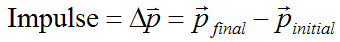In this case, we set the denominator equal to zero.

x2 + 2x – 8 = 0

(x + 4)(x – 2) = 0

x = –4 or x = 2

Since we cannot divide by zero, it means that there are two asymptotes; at x= -4 and x= 2.

• Problem two: Find the vertical asymptote of the following function: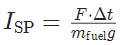Here are the calculations. Note that we start by checking the zeroes of the denominator:

x2 + 5x + 6 = 0

(x + 3)(x + 2) = 0

x = –3 or x = –2

Because it is impossible to divide by zero, it means that we have several vertical asymptotes at: x= -3 and x=-2.

• Problem 3: Find the vertical asymptote of the following function: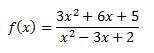Here, we start by solving the denominator equal to zero, as shown below.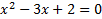Then, we go ahead to solve the quadratic by factoring the trinomial. Have a look: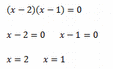There are two vertical asymptotes for this function: at x=2 and x=1.

Now, can you try more examples?

### Vertical Asymptote of Rational Functions.. Isn’t it Fun?

From this discussion, finding the vertical asymptote came out to be a fun activity. Well, you only need to understand the definition and the vertical asymptote rules. Then, practice using the examples provided above to grasp the concept well. Isn’t it fun?

At this point, we also need to say the truth: how to find vertical and horizontal asymptote is no simple task. You need to develop problem-solving skills. This makes it difficult for some students to complete their assignments on time. In other cases, you might have other engagements or find the deadline too tight to complete the assignment. It really shouldn’t worry you if you’re having “I really need to do my calculus homework, but I have no time!” thoughts. No matter the reason for making it hard for you to complete the college vertical asymptote assignment, you should seek writing help.

Writing help for college students is offered by expert writers who understand what is an asymptote and how to calculate both vertical and horizontal asymptotes. As the experts prepare the assignment for you, take the time to hone your skills for solving asymptotes.

Do not let finding horizontal and vertical asymptotes stress you: this guide is all you need to solve the problems like a pro!

Let's stand with the heroes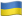As Putin continues killing civilians, bombing kindergartens, and threatening WWIII, Ukraine fights for the world's peaceful future.

Ukraine Live Updateshttps://war.ukraine.ua/
Donate Directly to Ukraine